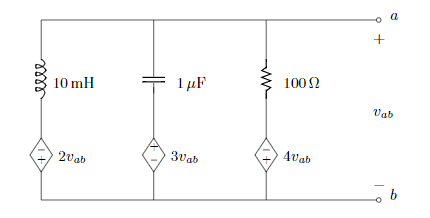# Thevenin Equivalent of AC RLC Circuit w/ Dependent Voltage

• Engineering
• YellowBelliedMarmot

## Homework StatementFind Vth and Zth.
frequency = 2kHz

## Homework Equations

w = 2 * (pi) * f
ZL = j w L
ZC = -j / (w * C)
V = I*Z
ZR = R

## The Attempt at a Solution

At first I tried using nodal analysis which I receive the value V = 0 v. I believe this is because this circuit only has dependent voltage sources. Therefore, the Vth is just 0 v.

10mH ---> j125.7
1uF ---> -j79.6
100 ohms ---> 100
w = 2*(pi)*2000 = 12566.37

Essentially, I am stuck. I have some theories as to what I can do though. I remember from DC circuit analysis that we can either add a 1v or a 1A (the number is arbitrary) source between terminals a and b, but I'm not sure what to do beyond that. Could I add a 1A (i.e. 1*cos(wt)) between the terminals, and then do nodal analysis? Then after that, would I take that voltage and divide it by the 1A (1cos(wt)) AC source to get the Zth? And as I mentioned above, wouldn't the thevenin equivalent of this circuit not even have a Vth since there's no independent sources?

•